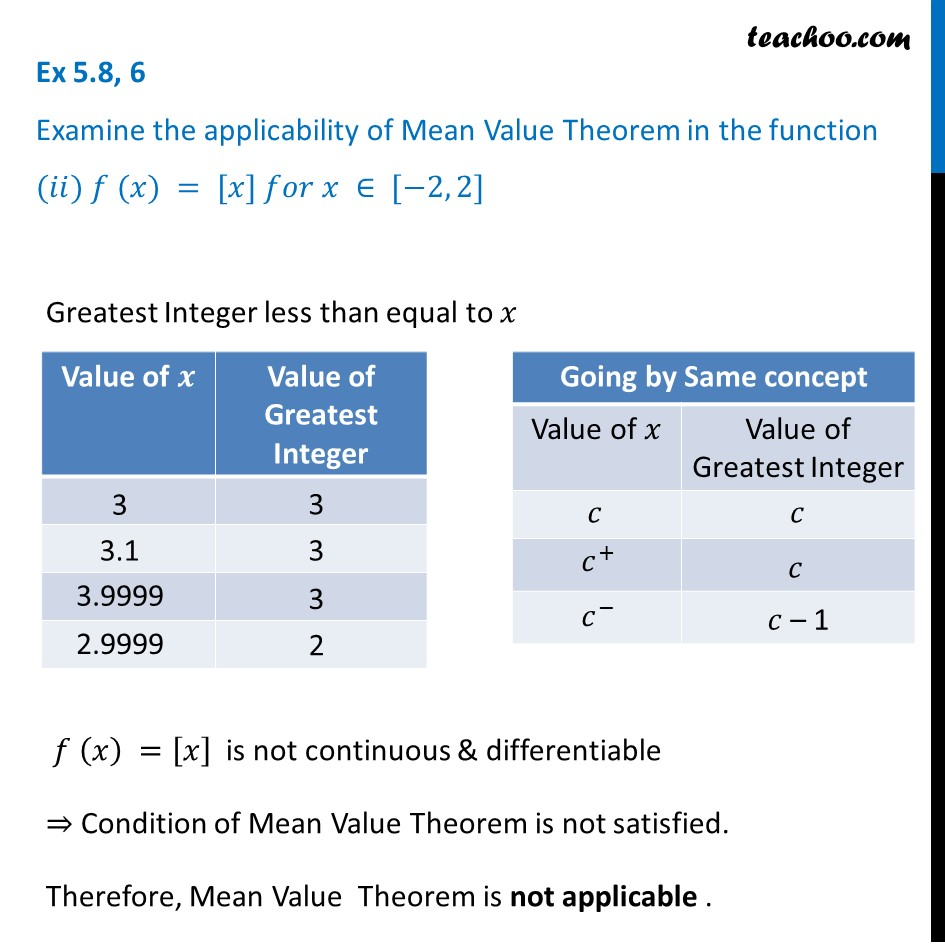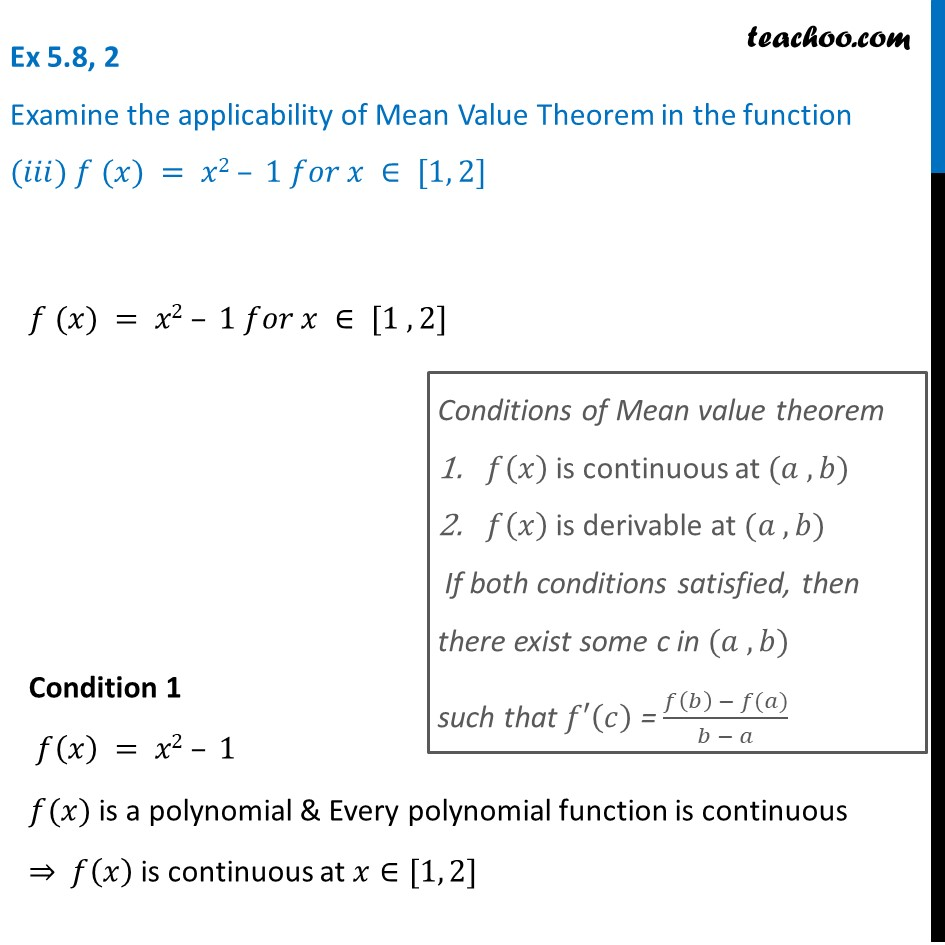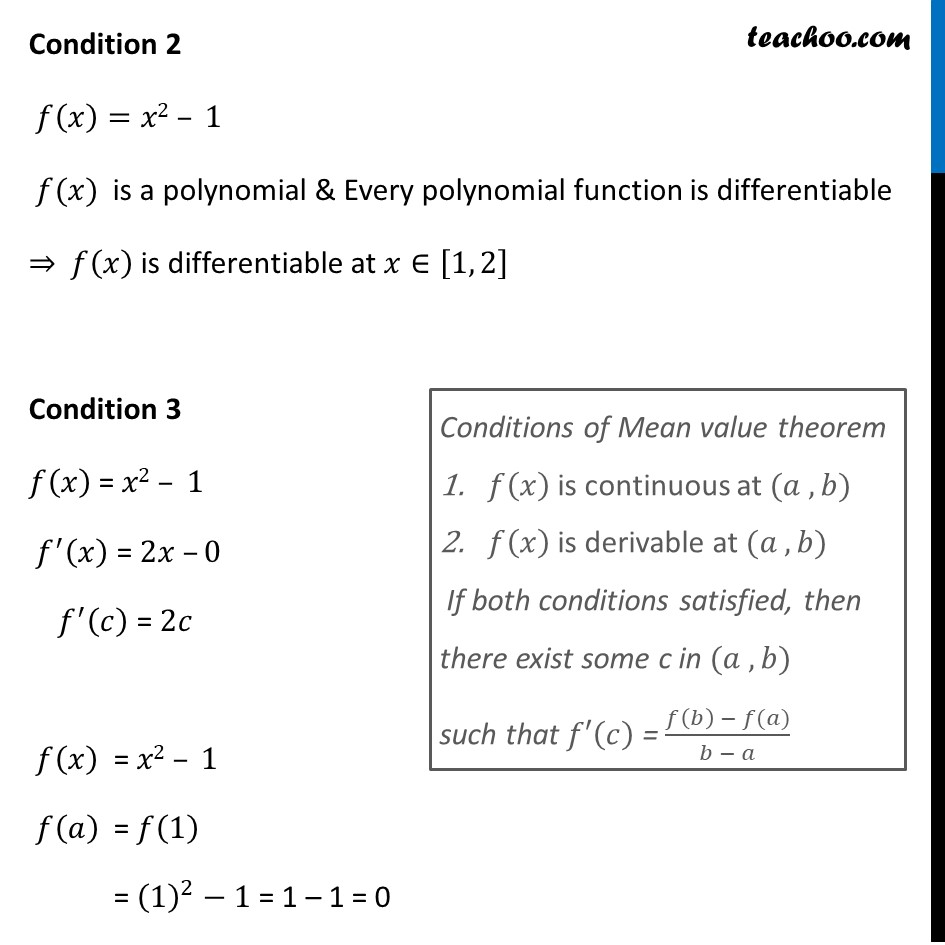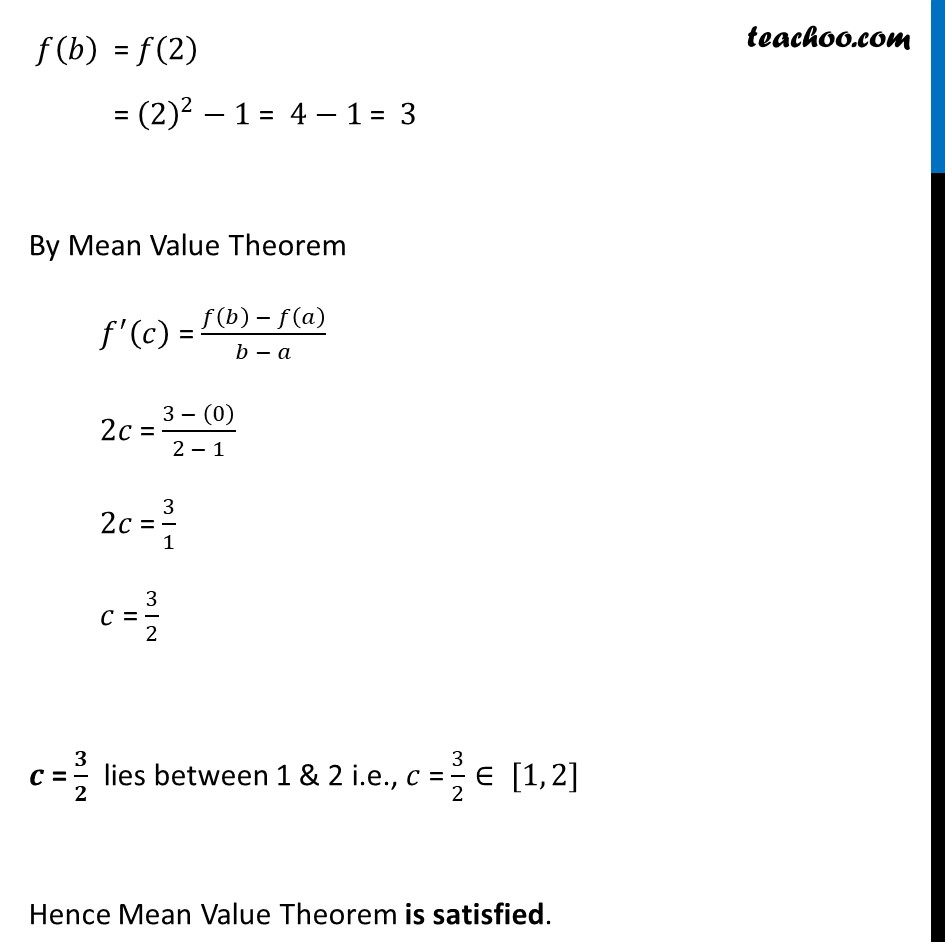Verify Mean Value Theorem

Chapter 5 Class 12 Continuity and Differentiability
Concept wiseIntroducing your new favourite teacher - Teachoo Black, at only ₹83 per month

### Transcript

Ex 5.8, 6 Examine the applicability of Mean Value Theorem in the function (𝑖) 𝑓 (𝑥) = [𝑥] 𝑓𝑜𝑟 𝑥 ∈ [5, 9]Greatest Integer less than equal to 𝑥 𝑓 (𝑥) =[𝑥] is not continuous & differentiable ⇒ Condition of Mean Value Theorem is not satisfied. Therefore, Mean Value Theorem is not applicable . Ex 5.8, 6 Examine the applicability of Mean Value Theorem in the function (𝑖𝑖) 𝑓 (𝑥) = [𝑥] 𝑓𝑜𝑟 𝑥 ∈ [−2, 2]Greatest Integer less than equal to 𝑥 𝑓 (𝑥) =[𝑥] is not continuous & differentiable ⇒ Condition of Mean Value Theorem is not satisfied. Therefore, Mean Value Theorem is not applicable . Ex 5.8, 2 Examine the applicability of Mean Value Theorem in the function (𝑖𝑖𝑖) 𝑓 (𝑥) = 𝑥2 – 1 𝑓𝑜𝑟 𝑥 ∈ [1, 2]𝑓 (𝑥) = 𝑥2 – 1 𝑓𝑜𝑟 𝑥 ∈ [1 , 2] Condition 1 𝑓(𝑥) = 𝑥2 – 1 𝑓(𝑥) is a polynomial & Every polynomial function is continuous ⇒ 𝑓(𝑥) is continuous at 𝑥∈[1, 2]Conditions of Mean value theorem 𝑓(𝑥) is continuous at (𝑎 , 𝑏) 𝑓(𝑥) is derivable at (𝑎 , 𝑏) If both conditions satisfied, then there exist some c in (𝑎 , 𝑏) such that 𝑓′(𝑐) = (𝑓(𝑏) − 𝑓(𝑎))/(𝑏 − 𝑎) 𝑓(𝑏)" = " 𝑓(2) = (2)^2−1 = 4−1 = 3 By Mean Value Theorem 𝑓^′ (𝑐) = (𝑓(𝑏) − 𝑓(𝑎))/(𝑏 − 𝑎) 2𝑐 = (3 − (0))/(2 − 1) 2𝑐 = 3/1 𝑐 = 3/2 𝒄 = 𝟑/𝟐 lies between 1 & 2 i.e., 𝑐 = 3/2∈ [1, 2] Hence Mean Value Theorem is satisfied. Ex 5.8, 6 Examine the applicability of Mean Value Theorem in the function (𝑖) 𝑓 (𝑥) = [𝑥] 𝑓𝑜𝑟 𝑥 ∈ [5, 9] Greatest Integer less than equal to 𝑥 𝑓 𝑥﷯ = 𝑥﷯ is not continuous & differentiable ⇒ Condition of Mean Value Theorem is not satisfied. Therefore, Mean Value Theorem is not applicable . Ex 5.8, 6 Examine the applicability of Mean Value Theorem in the function (𝑖𝑖) 𝑓 (𝑥) = [𝑥] 𝑓𝑜𝑟 𝑥 ∈ [−2, 2] Greatest Integer less than equal to 𝑥 𝑓 𝑥﷯ = 𝑥﷯ is not continuous & differentiable ⇒ Condition of Mean Value Theorem is not satisfied. Therefore, Mean Value Theorem is not applicable . Ex 5.8, 2 Examine the applicability of Mean Value Theorem in the function (𝑖𝑖𝑖) 𝑓 (𝑥) = 𝑥2 – 1 𝑓𝑜𝑟 𝑥 ∈ [1, 2] 𝑓 (𝑥) = 𝑥2 – 1 𝑓𝑜𝑟 𝑥 ∈ [1 , 2] Condition 1 𝑓 𝑥﷯ = 𝑥2 – 1 𝑓(𝑥) is a polynomial & Every polynomial function is continuous ⇒ 𝑓 𝑥﷯ is continuous at 𝑥∈[1, 2] Condition 2 𝑓 𝑥﷯=𝑥2 – 1 𝑓(𝑥) is a polynomial & Every polynomial function is differentiable ⇒ 𝑓 𝑥﷯ is differentiable at 𝑥∈ 1, 2﷯ Condition 3 𝑓 𝑥﷯ = 𝑥2 – 1 𝑓﷮′﷯ 𝑥﷯ = 2𝑥 –0 𝑓﷮′﷯ 𝑐﷯ = 2𝑐 𝑓 𝑥﷯ = 𝑥2 – 1 𝑓 𝑎﷯ = 𝑓 1﷯ = 1﷯﷮2﷯−1 = 1 – 1 = 0 𝑓 𝑏﷯ = 𝑓 2﷯ = 2﷯﷮2﷯−1 = 4−1 = 3 By Mean Value Theorem 𝑓﷮′﷯ 𝑐﷯ = 𝑓 𝑏﷯ − 𝑓 𝑎﷯﷮𝑏 − 𝑎﷯ 2𝑐 = 3 − 0﷯﷮2 − 1﷯ 2𝑐 = 3﷮1﷯ 𝑐 = 3﷮2﷯ 𝒄 = 𝟑﷮𝟐﷯ lies between 1 & 2 i.e., 𝑐 = 3﷮2﷯∈ [1, 2] Hence Mean Value Theorem is satisfied.